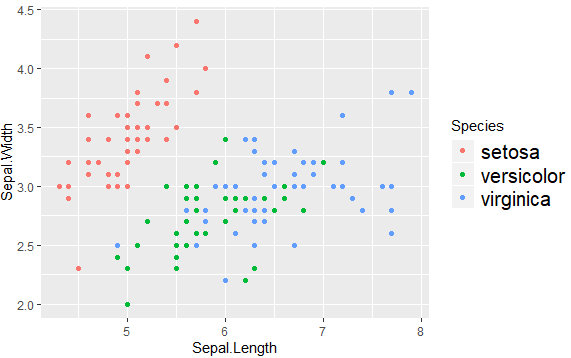# increase legend font size ggplot2

0 votes
1 view

Is there a way to increase the font size in ggplot2? I think I need to specify something like legend.key.width = unit(2, "line") in the theme function, but that is used to adjust the keys in legends, not the font sizes. Thanks!

## 1 Answer

0 votes
by (25.4k points)

To increase the legend font size in ggplot2, you can add the following to your plot:

theme(legend.text=element_text(size=X))

Where

X is the size.

For example:

ggplot(data = iris,

aes(x = Sepal.Length, y = Sepal.Width,col = Species, label = Species)) +

geom_point() +

theme(legend.text=element_text(size=15))

Output:0 votes
1 answer
0 votes
1 answer
0 votes
1 answer
0 votes
1 answer
0 votes
1 answer